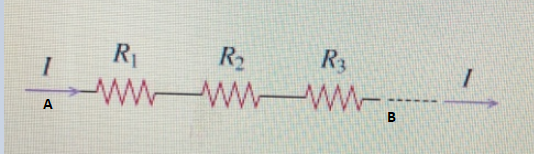# Problem: For the set-up shown, find the equivalent resistance between points A and B.

###### FREE Expert Solution

Equivalent resistance for series resistors:

$\overline{){{\mathbf{R}}}_{\mathbf{e}\mathbf{q}}{\mathbf{=}}{{\mathbf{R}}}_{{\mathbf{1}}}{\mathbf{+}}{{\mathbf{R}}}_{{\mathbf{2}}}}$

The resistors given are in series.###### Problem Details

For the set-up shown, find the equivalent resistance between points A and B.